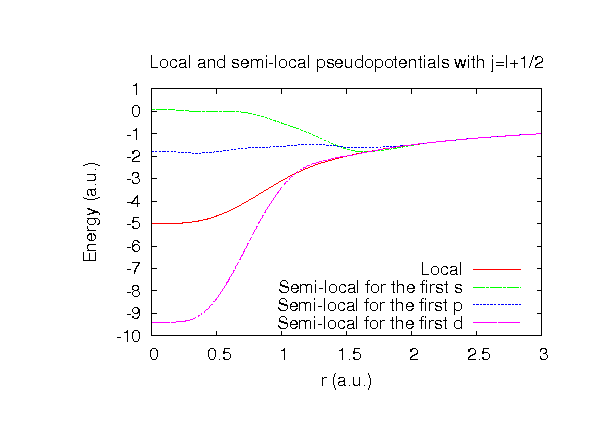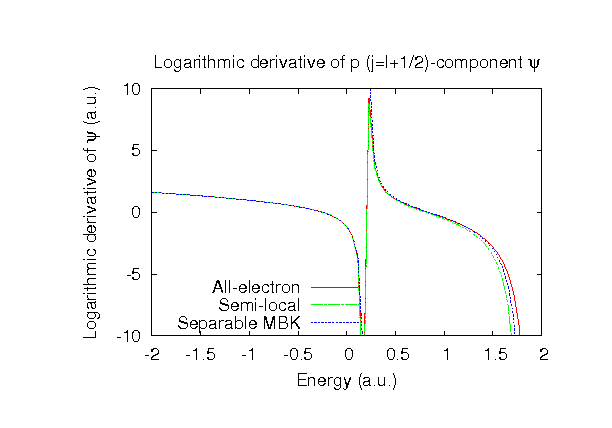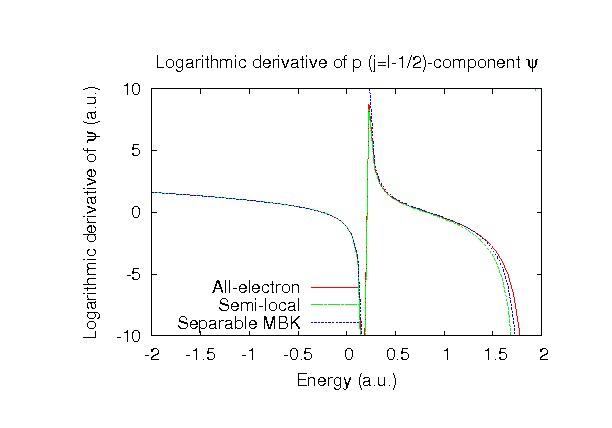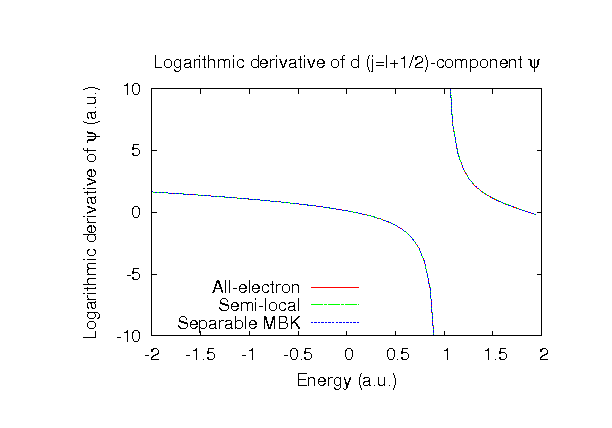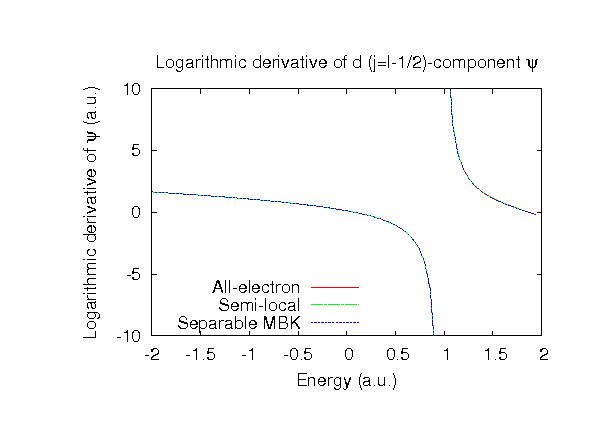### Fully relativistic pseudopotentials

Fully relativistic pseudopotentials generated by the MBK (PRB 47, 6728 (1993)) scheme within LDA (CA13) and GGA (PBE13) which contain a partial core correction and fully relativistic effects including spin-orbit coupling.

### Pseudo-atomic orbitals

The number below the symbol means a cutoff radius (a.u.) of the confinement potential. These file includes fifteen radial parts for each angular momentum quantum number l (=0,1,2,3,4). The basis functions were generated by variationally optimizing the corresponding primitive basis functions in the single atom and the dimer molecule. The input file used for the orbital optimization can be found at Al_opt.dat and Al2_opt.dat. Since Al_CA13.vps and Al_PBE13.vps include the 3s and 3p states (3 electrons) as the valence states, the minimal basis set is Al*.*-s1p1. Our recommendation for the choice of cutoff radius of basis functions is that Al7.0.pao is enough for bulks, but Al8.0.pao or Al9.0.pao is preferable for molecular systems.

### Benchmark calculations by the PBE13 pseudopotential with the various basis functions

(1) Calculation of the total energy as a function of lattice constant in the fcc structure, where the total energy is plotted relative to the minimum energy for each case. a0 and B0 are the equilibrium lattice constant and bulk modulus obtained by fitting to the Murnaghan equation of state. The difference between Al7.0-s3p3d2 and Al7.0-s4p4d2 in the total energy at the minimum point is 0.003 eV/atom. An input file used for the OpenMX calculations can be found at Alfcc-EvsV.dat . For comparison the result by the Wien2k code is also shown, where the calculation was performed by default setting in the Ver. 10.1 of Wien2k except for the use of RMT x KMAX of 12.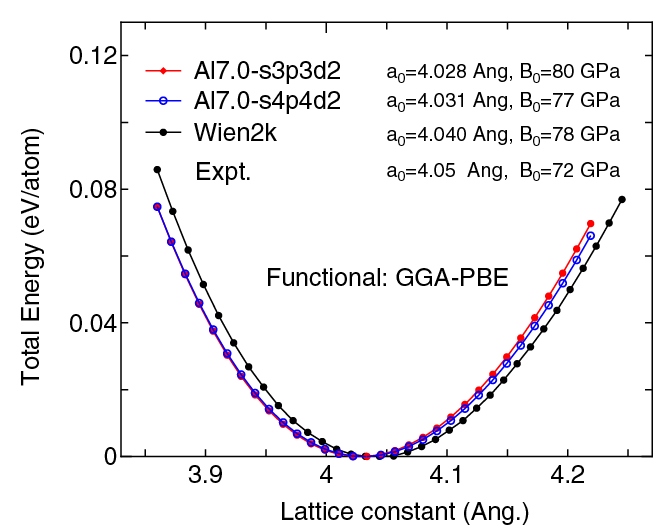(2) Calculations of the band dispersion in the fcc structure, where the non-spin polarized collinear calculation with the lattice constant of 4.05 Ang. was performed using Al_PBE13.vps and Al7.0-s3p3d2, and the origin of the energy is taken to be the Fermi level. The input file used for the OpenMX calculations can be found at Alfcc-Band.dat . For comparison the result by the Wien2k code is also shown, where the calculation was performed by default setting in the Ver. 10.1 of Wien2k except for the use of RMT x KMAX of 12.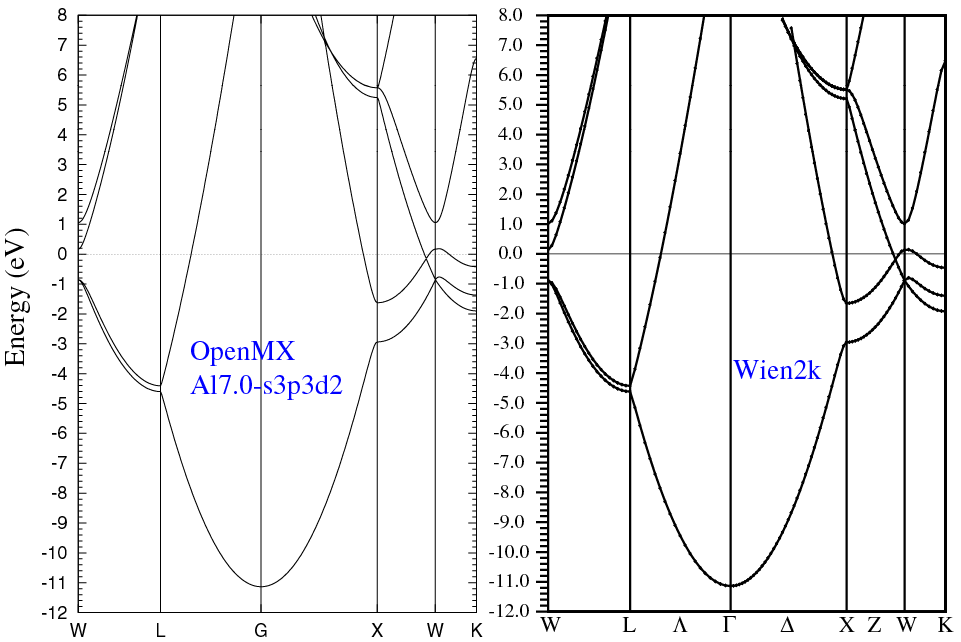(3) Calculations of a Al dimer molecule, where Al_PBE13.vps, Al9.0-s3p3d2, and Al9.0-s4p4d2 were used. The input files used for the OpenMX calculations can be found at Al2.dat , Al_cp.dat , and Al.dat ,

 Equilibrium bond length (Ang.) Atomization energy (eV) Atomization energy (couterpoise corrected) (eV) Al9.0-s3p3d2 2.641 1.818 1.744 Al9.0-s4p4d2 2.640 1.820 1.745 Other calc. 2.636 a Expt. 2.466 b

a J. G. O. Ojwang et al., J. Chem. Phys. 129, 244506 (2008).
b K.P. Huber and G. Herzberg, "Constants of Diatomic Molecules" (data prepared by J.W. Gallagher and R.D. Johnson, III) in NIST Chemistry WebBook, NIST Standard Reference Database Number 69, Eds. P.J. Linstrom and W.G. Mallard, National Institute of Standards and Technology, Gaithersburg MD, 20899, http://webbook.nist.gov, (retrieved January 12, 2011).

### Supplementary information for the GGA (PBE13) pseudopotential